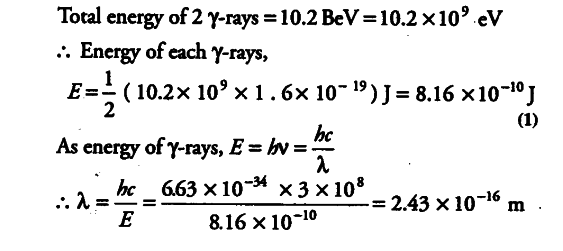# In an accelerator experiment on high energy

In an accelerator experiment on high energy collisions of electrons with positrons, a certain event is interpreted as annihilation of an electron-positron pair of total energy 10.2 BeV into two $\gamma$-rays of equal energy. What is the wavelength associated with each $\gamma$-ray?